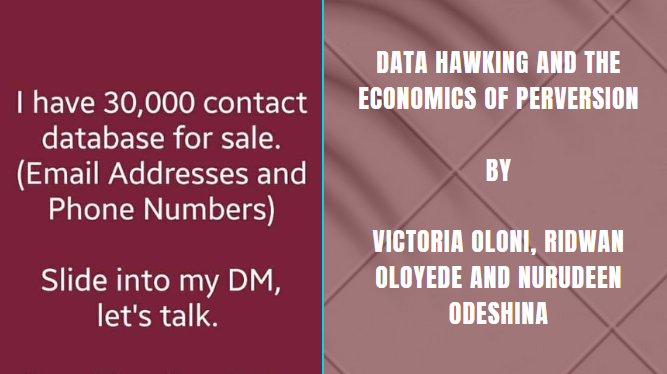# Attacking the Weakness from Related RSA Public Keys

RSA by itself is a strong public key cryptosystem. All the known attacks against RSA emanates from poor implementation and usage of the system. One of such is poor random number generation which is a topic for another day. The attack we are focusing on is as a result of a weakness created when two related public keys exist.

The cryptosystem is built around modular arithmetic and factorisation so let’s talk a little bit about the math behind the attack because modern cryptography is purely mathematics. To keep things simple, I will mention only the the formula that is relevant to this attack alone.

The modulus (N) = p*q

The modulus (N) is the public key mainly used to encrypt messages in the public key cryptosystem and it is usually a very large prime number.

Note that N is the public key so it is always known by the general public.

p and q are two large primes randomly selected during the key generation process and it is extremely difficult and usually computationally infeasible to deduce their values from N. It is very important that the selection is random and it is not repeated.

A problem arises when we have two public keys N1 and N2 where p is a match in both N1, and N2. We say the keys are related.

Remember N = p*q

So we now have N1=p*q1 and N2=p*q2

Notice how p remains the same in both equations.

To cut the story short, the Greatest Common Divisor of N1 and N2 is p because p is the common prime factor between both of them.

Remember N1 and N2 are public keys so they are already known and their GCD can be calculated.

GCD(N1,N2)=p iff N1=p*q1 and N2=p*q2

When we find the value of p, we can substitute it into the equation to find the value of q1 and q2 such that

q1=N1/p and q2=N2/p

So we now know p and q for both public keys (N1 and N2). These are the two primes that were used to create the public key.

This means that we can now recreate the private key for each of the related public keys 😎

I will not talk about the key generation process but the script provided here automates the mathematical steps and also recreates the private key.

The user only needs to provide decimal values of the two related public keys as input.

Below are two related 1024 bit public keys you can experiment with (both in decimal value). If you are curious enough, you can use other tools (openssl) to verify the correctness of the private key you have gotten.

N1 = 59881702787163980803850570560437348485136246918937202746322203477429473577086346121469004621774079512738262205051918860627998386091773164249718847697709064883106911212788793617698098194551759454893963980781813517132328214857750915758856604048242776963585140119480045424332242756394234210893445718206047563227

N2 = 85581272086017633255498371589254822498690330955952204013887413323132878927687189282378160511863636303991329497441857501898705898857334883406104166447609787381340385229115619878104911989700389017660383746810086088725923143989846098214106737129761697403453817914183890294812008556035991874368314790910047246813

5 1 vote
Article Rating
Subscribe
Notify ofInline Feedbacks
Prev
Data hawking and the economics of perversion## Data hawking and the economics of perversion

Data hawking and the economics of perversion By Victoria Oloni, Ridwan Oloyede

Next
What To Do After You Have Been Hacked## What To Do After You Have Been Hacked

There was a time when Whatsapp was very easy hacker target before it was

##### You May Also Like0